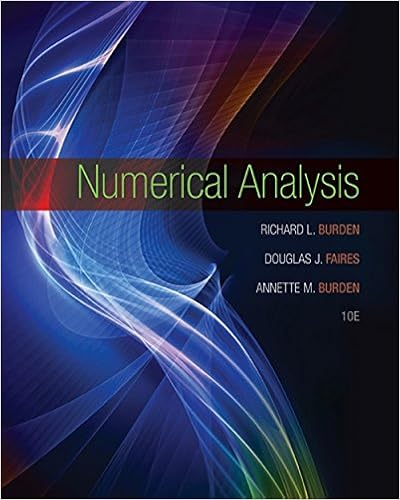# 58 draw a graph of the following 2 1 y x section 32 4

• Notes
• 32
• 100% (22) 22 out of 22 people found this document helpful

This preview shows page 31 - 32 out of 32 pages.

##### We have textbook solutions for you!
The document you are viewing contains questions related to this textbook.The document you are viewing contains questions related to this textbook.
Chapter 4 / Exercise 13
Numerical Analysis
Burden/FairesExpert Verified
58) Draw a graph of the following: 2 1 y x = - + . (Section 3.2) -4 -4 -4 -4 -2 -2 -2 -2 2 4 -4 -4 -4 -4 -2 -2 -2 -2 2 4 x y x y 0 3 1 2 2 1 3 2 4 3
##### We have textbook solutions for you!
The document you are viewing contains questions related to this textbook.The document you are viewing contains questions related to this textbook.
Chapter 4 / Exercise 13
Numerical Analysis
Burden/FairesExpert Verified
Math 012 -- KEY 60 Practice Problems for the Final Exam 32 59) Simplify: 2 3 9 3 12 15 27 64 x y x y - - . (Sections 5.2 and 7.2) 2 2 3 9 9 3 3 12 15 6 2 6 3 2 4 3 6 4 27 27 64 64 27 64 9 16 x y x x y y x y x y - -   =     = = 60) For what values of x is the following expression undefined? 3 2 2 3 9 12 x x x x - + - (Section 6.1) A rational expression is undefined at any value of x that would make the denominator equal zero. We factor the denominator: ( ) ( )( ) 3 2 2 2 2 3 9 12 3 3 4 2 3 4 1 x x x x x x x x x x x x - - = + - + - - = + - This rational expression will be undefined if x = 0, -4, or 1.
•••